# Trading Using Machine Learning In Python Part-2### Continued:

At the end of my last blog, I had asked a few questions. Now, I will answer them all at the same time. I will also discuss a way to detect the regime/trend in the market without training the algorithm for trends. But before we go ahead, please use a fix to fetch the data from Google to run the code below.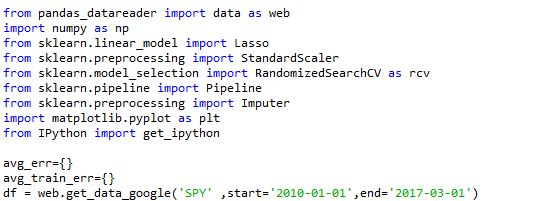Is the equation over-fitting?

This was the first question I had asked. To know if your data is overfitting or not, the best way to test it would be to check the prediction error that the algorithm makes in the train and test data.

To do this, we will have to add a small piece of code to the already written code.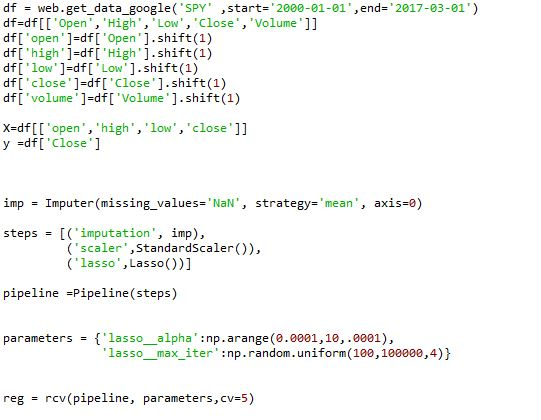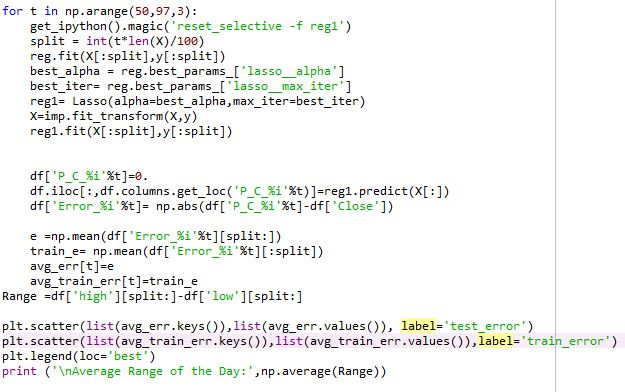First, let me begin my explanation by apologizing for breaking the norms: going beyond the 80 column mark.

Second, if we run this piece of code, then the output would look something like this.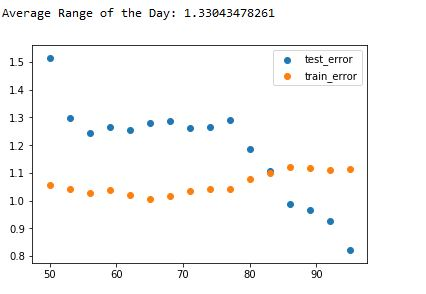Our algorithm is doing better in the test data compared to the train data. This observation in itself is a red flag. There are a few reasons why our test data error could be better than the train data error:
1. If the train data had a greater volatility (Daily range) compared to the test set, then the prediction would also exhibit greater volatility.
2. If there was an inherent trend in the market that helped the algo make better predictions.
Now, let us check which of these cases is true. If the range of the test data was less than the train data, then the error should have decreased after passing more than 80% of the data as a train set, but it increases.

Next, to check if there was a trend, let us pass more data from a different time period.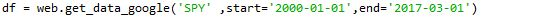If we run the code the result would look like this: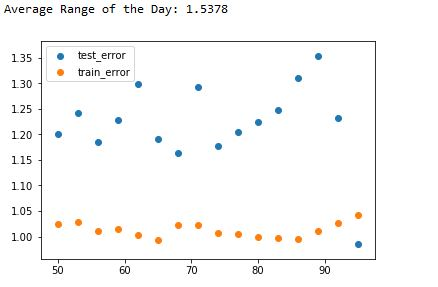So, giving more data did not make your algorithm work better, but it made it worse. In a time series data, the inherent trend plays a very important role in the performance of the algorithm on the test data. As we saw above it can yield better than expected results sometimes. The main reason why our algo was doing so well was the test data was sticking to the main pattern observed in the train data.

So, if our algorithm can detect underlying the trend and use a strategy for that trend, then it should give better results. I will explain this in more detail:

1. Can the machine learning algorithm detect the inherent trend or market phase (bull/bear/sideways/breakout/panic).
2. Can the database be trimmed in a way to train different algos for different situations
The answer to both the questions is a YES!

We can divide the market into different regimes and then use these signals to trim the data and train different algorithms for these datasets. To achieve this, I choose to use an unsupervised machine learning algorithm.

From here on, this blog will be dedicated to creating an algorithm that can detect the inherent trend in the market without explicitly training for it.

First, let us import the necessary libraries.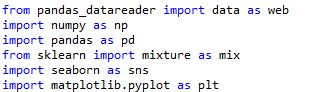Then we fetch the OHLC data from Google and shift it by one day to train the algorithm only on the past data.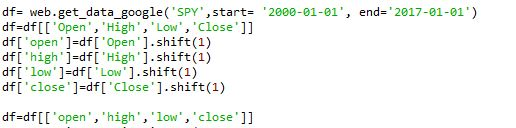Then drop all the NaN.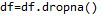Next, we will instantiate an unsupervised machine learning algorithm using the ‘Gaussian mixture’ model from sklearn.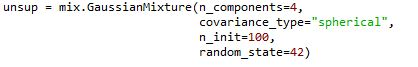In the above code, I created an unsupervised-algo that will divide the market into 4 regimes, based on the criterion of its own choosing. We have not provided any train dataset with labels like in the previous blog.

Next, we will fit the data and predict the regimes. Then we will be storing these regime predictions in a new variable called regime.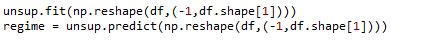Now let us calculate the returns of the day.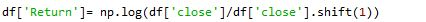Then, create a dataframe called Regimes which will have the OHLC and Return values along with the corresponding regime classification.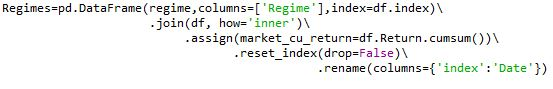After this, let us create a list called ‘order’ that has the values corresponding to the regime classification, and then plot these values to see how well the algo has classified.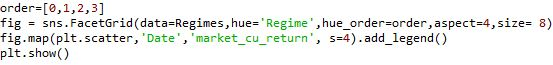The final regime differentiation would look like this: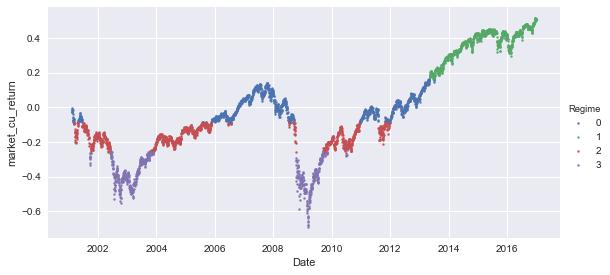This graph looks pretty good to me. Without actually looking at the factors based on which the classification was done, we can conclude a few things just by looking at the chart.

1. The red zone is the low volatility or the sideways zone
2. The purple zone is high volatility zone or panic zone.
3. The green zone is a breakout zone.
4. The blue zone: Not entirely sure but let us find out.
Use the code below to print the relevant data for each regime.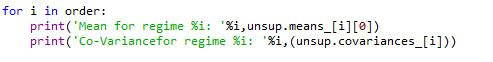The output would look like this: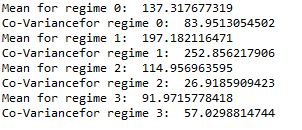The data can be inferred as follows:

1. Regime 0: Low mean and High covariance.
2. Regime 1: High mean and High covariance.
3. Regime 2: High mean and Low covariance.
4. Regime 3: Low mean and Low covariance.
So far, we have seen how we can split the market into various regimes. But the question of implementing a successful strategy is still unanswered. If you want to learn how to code a machine learning trading strategy then your choice is simple:

To rephrase Morpheus,

This is your last chance. After this, there is no turning back. You take the blue pill—the story ends, you wake up in your bed and believe that you can trade manually. You take the red pill—you stay in the Algoland, and I show you how deep the rabbit hole goes.

Remember: all I'm offering is the truth. Nothing more.

### Next Step

At this moment, AI and Machine Learning have already progressed enough and they can predict stock prices with a great level of accuracy. So what makes it possible? read our post on 'Machine Learning For Trading – How To Predict Stock Prices Using Regression?' to know more.